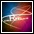blog.Ring.idv.tw

# ActionScript

##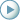「loadVariables」vs.「LoadVars」(二)

```var data_lv:LoadVars = new LoadVars();
{
if(success)
{
trace(this.ok);
}else{
trace("Failed!");
}
}
```

```Loaded!
1
```

```this.loadVariables("data.txt");
this.onData = function()
{
if(this.ok == undefined)
{
}else{
trace(this.ok);
}
}
```

```Loading...
1
```

setInterval

```this.loadVariables("data.txt");
{
if(_root.ok == undefined)
{
}else{
trace(_root.ok);
clearInterval(param_interval);
}
}
```

```Loading...
1
```

MovieClip.onEnterFrame

```this.loadVariables("data.txt");
this.onEnterFrame = function()
{
if(this.ok == undefined)
{
}else{
trace(this.ok);
delete this.onEnterFrame;
}
}
```

```Loading...
1
```

##「loadVariables」vs.「LoadVars」(一)

```var foo = 10;
var bar = 20;

data_lv.foo = 10;
```

```Error opening URL 'http://localhost/loadVariables.jsp?foo=10&bar=20'
```

```var foo = 10;
var bar = 20;

this.createEmptyMovieClip("data_mc",1);
data_mc.foo = 30;
```

```Error opening URL 'http://localhost/loadVariables.jsp?foo=30'
```

##從元件庫(Library)附加你的元件(一)-「attachMovie()」

「attachMovie()」-從字面上所表徵的意思，「attach」可以解釋為「貼上」或「附加」等意思，加上後面的「Movie」便知大意為「附加上你的影片片段」，也就是說，當我們想要從元件庫(Library)附加上「元件」時，即可利用這個方法來幫助我們達成。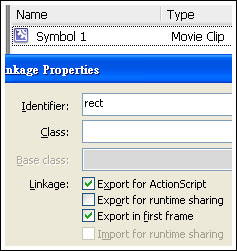```trace(this);
```

Parameters

id:String — 對應元件庫中的識別名稱。

name:String — 給予它一個「實體名稱(instance name)」。

depth:Number — 深度值，可參考「Layer」、「Level」和「Depth」之間的三角關係(四)

initObject:Object (optional) — 在Flash Player 6之後才被支援的參數，可用來初始化此實體的一些屬性。

```_root.attachMovie("rect","rect_mc",1);
```

```_level0.rect_mc
```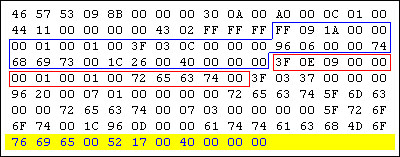```var obj = new Object();
obj._x = 50;
obj._y = 50;
_root.attachMovie("rect","rect_mc",_root.getNextHighestDepth(),obj);
```

```_root.attachMovie("rect","rect_mc",_root.getNextHighestDepth());
rect_mc._x = 50;
rect_mc._y = 50;
```

##「Number()」vs.「parseInt()」(一)-效率測試

「Number()」:

```function test()
{
for(var j = 0 ; j < 100000; j++)
{
Number("10000");
}
}
var start = getTimer();
test();
trace(getTimer()-start);
```

```202
```

「parseInt()」:

```function test()
{
for(var j = 0 ; j < 100000; j++)
{
parseInt("10000");
}
}
var start = getTimer();
test();
trace(getTimer()-start);
```

```314
```

```var a_num = Number("1.5");
var b_num = parseInt("1.5");
```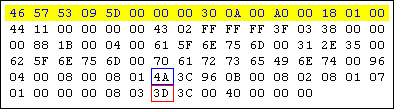##「Target Path」命中你的目標實體(四)

「_root」絕對路徑 vs. 「_parent」相對路徑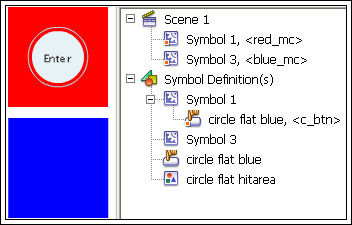```on(release)
{
trace(this);
}
```

```_level0.red_mc
```

```on(release)
{
_parent.blue_mc._alpha = 50;
}
```

```on(release)
{
_root.blue_mc._alpha = 50;
}
```

「_root」和「_parent」的取捨